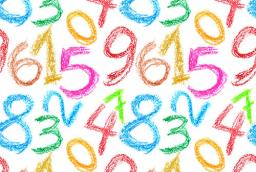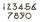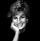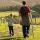# Unknown number

I think the number: Its half is 16 bigger than his quarter. I think the number N . ..

N =  64

### Step-by-step explanation:

N/2=16+N/4

N = 64

Our simple equation calculator calculates it.Did you find an error or inaccuracy? Feel free to write us. Thank you!Tips to related online calculators
Do you have a linear equation or system of equations and looking for its solution? Or do you have a quadratic equation?

## Related math problems and questions:

• Unknown number 16My number's tens is three times more then ones My number's ones is twice the number of thousands and my number's hundreds is half the number of of tens. I have two ones. which number am I?
• Unknown number 24I think the number: a - is the same as the square area that has the 12th circumference. What is this number? b - its half is 7 times bigger than its quarter. Is this the number?
• Unknown number 716% of the unknown number is by 21 less than unknown number itself. Determine the natural unknown number.
• Unknown numberFind the unknown number equal to a quarter of a fifth of a number, which is by 152 more than an unknown number.
• Unknown numberSamuel wrote unknown number. Then he had add 200000 to the number and the result multiply by three. When it calculated he was surprised, because the result would have received anyway, if write digit to the end of original number. Find unknown number.
• Unknown number 5I think of an unknown number. If we enlarge it five times, then subtract 3, and the result decreases by 75%, we get one greater than the number. What number am I thinking of?
• SixteenSixteen minus the quotient of 12 and what number is thirteen?
• DivisionWhich number in division 16 give 12 and the rest 3?
• Number unknownAdela thought the two-digit number, she added it to its ten times and got 407. What number does she think?
• NumberWhat number do I think? The third is 6, and a half is 2 more than its quarter.
• My fatherMy father has a big farm. 6/8 of it were planted with mango trees, 1/2 of the remainder are guava trees and the rest 10 trees are santol trees. What is the number of all trees?
• UN 1If we add to an unknown number his quarter, we get 210. Identify unknown number.
• After 16 yearsAfter 16 years will Dana be five times older as she was four years ago. After how many years will Dana celebrate her 16th birthday?
• Brick weightThe brick weighs 3 kg and a half-brick. How much does one brick weigh?
• NumberDetermine unknown number if you know that difference between five times and triple of number is 42.Daddy is a 46-year-old, and his son is at the age of 16. When (which year) was/will daddy five times more years than his son?Number first increased by 30%, then by 1/5. What percentage we've increased the original number?